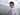# 22. 括号生成

March 17, 2020

## 错误解法：

``````    func generateParenthesis(n int) []string {
var res []string
if n < 1 {
return res
}
if n == 1 {
res = append(res, "()")
return res
}
temps := generateParenthesis(n-1)
for _, item := range temps {
a := "(" + item + ")"
res = append(res, a)
b := "()" + item
if a != b {
res = append(res, b)
}
c := item + "()"
if a!=c && b!=c {
res = append(res, c)
}
}
return res
}``````

### 正确解法：

``````    func generateParenthesis(n int) []string {
return generator(n, 0, "")
}

func generator(left, right int, s string) (res []string) {
if left == 0 && right == 0 {
res = append(res, s)
return
}
if left > 0 {
res = generator(left - 1, right + 1, s + "(")
}
if right > 0 {
res = append(res, generator(left, right - 1, s + ")")...)
}

return res
}``````

1. 一个 ”(” 对应一个 ”)“。因此每增加一个 ”(”，”(” 的待使用量就减少 1，”)” 的待使用量就增加 1
2. 第一个字符一定是 ”(“。因此我们从 ”(” 开始迭代，每个 ”(” 的下一个字符，可能是 ”(“，也可能是 ”)“，需要基于各自剩余的待使用量来确定。